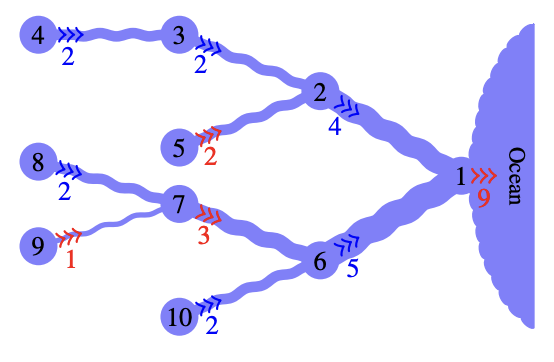시간 제한 메모리 제한 제출 정답 맞은 사람 정답 비율
4 초 512 MB 6 1 1 16.667%

## 문제

Last summer, Carla the Cartographer went on an expedition on behalf of the National Center for Positioning and Charting (the NCPC). The goal was to measure water flows of a river system far away in the north. However, the area is quite remote and Carla is not the adventurous type, so she was only able to measure the water flows in some of the locations. Carla is now worried that the NCPC will send her back to this wilderness next summer, so she consulted some algorithm experts (you) to see if it is possible to reconstruct the missing data.

The river system is represented by a rooted tree with n vertices numbered from 1 to n. The leaves of this tree are the sources, and the other vertices correspond to confluences (places where multiple rivers join together). Water flows from higher-numbered vertices to lower-numbered vertices. Vertex 1, the root of the tree, is the mouth of the river system, where it flows into the ocean. The water flow of a source can be any positive integer, while the water flow of a confluence is the sum of the water flows of its children. You will be given this tree along with the water flows at some of its vertices, and your task is to find the water flows at all the vertices or determine that this is impossibleFigure F.1: Illustration of Sample Input 1 and its solution. Water flows from left to right. The flows shown in red, from vertices 1, 5, 7 and 9, are not given but can be deduced to be 9, 2, 3 and 1 respectively

## 입력

The first line of input contains an integer n (2 ≤ n ≤ 3 · 105), the number of vertices in the tree. Then follows a line containing n − 1 integers p2, . . . , pn (1 ≤ pi < i), where pi is the number of the parent of vertex i.

Finally there is a line containing n integers a1, . . . , an (0 ≤ ai ≤ 109), where ai represents the water flow at vertex i. If ai is equal to 0, then the water flow at that vertex is unknown. Otherwise, ai is equal to the water flow at vertex i. Note that the upper bound 109 on ai does not apply to the unknown values, they can be any positive integer.

## 출력

If all the n water flows can be reconstructed uniquely, then output them in increasing order of vertex number. Otherwise, output “impossible”. Note that it is possible that there is no way of reconstructing the water flows (if the data provided by Carla is inconsistent somehow). In that case you should also output “impossible”.

## 예제 입력 1

10
1 2 3 2 1 6 7 7 6
0 4 2 2 0 5 0 2 0 2


## 예제 출력 1

9
4
2
2
2
5
3
2
1
2


## 예제 입력 2

5
1 2 2 1
4 0 0 0 1


## 예제 출력 2

impossible


## 예제 입력 3

4
1 1 1
3 2 1 0


## 예제 출력 3

impossible